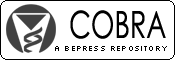## COBRA Preprint Series

#### Abstract

In this article we present new statistical methodology for the analysis of repeated measures of spatially correlated growth data. Our motivating application, a ten year study of height growth in a plantation of even-aged white spruce, presents several challenges for statistical analysis. Here, the growth measurements arise from an asymmetric distribution, with heavy tails, and thus standard longitudinal regression models based on a Gaussian error structure are not appropriate. We seek more flexibility for modeling both skewness and fat tails, and achieve this within the class of skew-elliptical distributions. Within this framework, robust space-time regression models are formulated using random effect growth curves, with coefficients arising from an underlying multivariate spatial process. Computational difficulties arise when data are collected at a large number of locations, and we consider two approaches for spatial modeling in the large data context. Both approaches are compared within the context of our application, and inference is conducted in a Bayesian framework, with implementation based on hybrid Monte Carlo.

#### Disciplines

Longitudinal Data Analysis and Time Series | Multivariate Analysis | Statistical Models

COinS